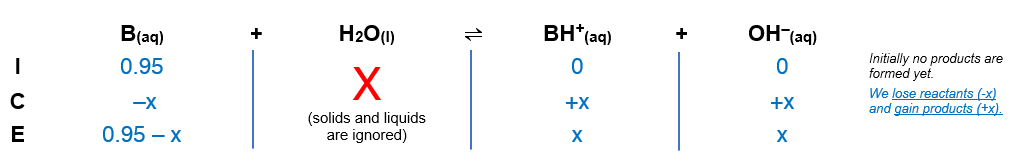# Problem: The pKb values for the dibasic base B are pKb1 = 2.1 and pKb2 = 7.5. Calculate the pH at each of the following points in the titration of 50.0 mL of a 0.95 M B(aq) with 0.95 M HCl(aq).(a) before addition of any HCl

###### FREE Expert Solution

We’re being asked to calculate the pH of a 0.95 M B

B (aq) + H2O (l) → BH+ (aq) + OH- (aq)

From this, we can construct an ICE table for the reaction of B in a solution. Remember that liquids are ignored in the ICE table.89% (301 ratings)###### Problem Details

The pKb values for the dibasic base B are pKb1 = 2.1 and pKb2 = 7.5.

Calculate the pH at each of the following points in the titration of 50.0 mL of a 0.95 M B(aq) with 0.95 M HCl(aq).

(a) before addition of any HCl